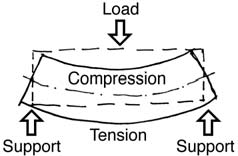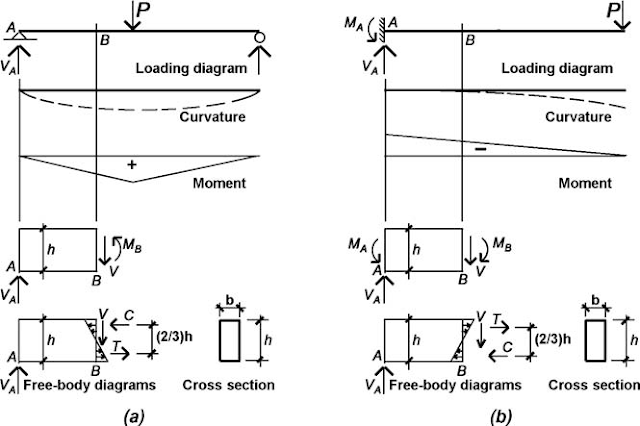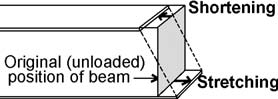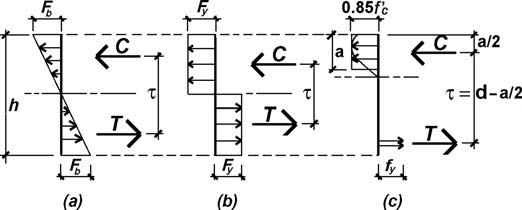# Bending Stress

Beams are stressed when they bend because the action of bending causes an elongation on one side, resulting in tension, and a shortening on the other side, resulting in compression. By exaggerating the curvature of the beam as it bends, this elongation and shortening can be visualized. Exactly where the tension and compression are depends on how the beam is loaded and how it is supported.FIGURE 1 Behavior of a simply supported beam
For simply supported beams with downward-acting loads (i.e., with gravity loads), the beam is stretched on the bottom (tension) and shortened on the top (compression) as shown in Figure 1 .
For cantilevered beams fixed at one end, with downward-acting loads, the beam is stretched on the top and shortened on the bottom ( Figure 2).FIGURE 2 Behavior of a cantilevered beam
For continuous beams spanning over several supports, the changing curvature causes the position of tension and compression zones to reverse a number of times over the length of the beam, as illustrated in Figure 3 .FIGURE 3 Behavior of a continuous beam
The relative position of tension and compression within the beam’s cross section is directly related to the sign of the bending moment at that cross section. As can be seen from Figure 4a, a counterclockwise moment on the right side of a freebody diagram is equivalent to a distribution of bending stress with compression on the top and tension on the bottom of the beam: “ positive ” bending (and “ positive ” bending moment). Figure 4b shows a free-body diagram cut through a cantilever beam with “ negative ” bending — that is, tension on the top and compression on the bottom corresponding to a clockwise moment as shown. The reversing curvature of a continuous beam, such as that shown in Figure 3 , corresponds precisely to a reversal in the sign of the bending moment. As shown in Figure 5, points of inflection (points where the curvature changes) always occur at points of zero moment.FIGURE 4 Comparison of “ positive ” and “ negative ” bending in (a) a simply supported beam and (b) a cantilevered beamFIGURE 5 Continuous beam showing correspondence of points of inflection (change from positive to negative curvature) and points of zero moment

Bending stresses within these beams can be computed if we assume that the stretching and shortening that take place at any cross section are linear; that is, a straight line connecting a stretched point with a shortened point on any cross sectional cut will accurately describe the shape of the beam throughout the entire cross section (Figure 6).FIGURE 6 Shortening and stretching (compression and tension) at a typical beam cross section

Three observations can be made once this assumption is accepted:
(1) maximum elongation and shortening occur at the top and bottom of the beam (the “extreme fibers” );
(2) a surface exists somewhere between the extreme fibers that is neither elongated nor shortened this “ plane ” is called the “ neutral axis ” or “neutral surface” ; and 3) strain can be defined as the elongation or shortening of any portion of the beam, divided by its original (unloaded) length. Since the original length is a constant, a strain diagram has the same shape as an “ elongation-shortening diagram. ”FIGURE 7 Elongation, strain, and stress diagrams for a linear, elastic material
For materials with linear stress-strain relationships (where stress equals strain times a constant modulus of elasticity ), a stress diagram will also have the same shape as the strain or “ elongation-shortening diagram. ” Figure 7 compares these diagram shapes for materials with linear stress-strain relationships. For materials with nonlinear stress-strain relationships, a stress diagram can be pieced together by plotting points from a stress-strain curve for the material. Thus, a steel beam stressed beyond its elastic region would have stress and strain distributions as shown in Figure 8. The elongation and shortening, shown in Figure 8a , and therefore the strain, shown in Figure 8b, are assumed to remain linear even when the stress, shown in Figure 8d through Figure 8f , becomes nonlinear.FIGURE 8 Elongation, strain, and stress diagrams for an elastic-plastic material such as steel showing (a) elongation and shortening of the actual material, (b) strain diagrams, (c) stress diagram at the point where the outer fi ber has just yielded, (d) stress diagram corresponding to strain just beyond the elastic limit, (e) stress diagram corresponding to continued strain beyond the elastic limit, (f) stress diagram corresponding to the plastic moment (where the entire cross section has yielded), and (g) stress-strain diagram

In Figure 8c , the stresses at the extreme fibers of the cross section just reach the limit of elastic behavior (with stress, σy ), which corresponds to the so-called elastic moment , Me . In Figure 8f , the strain at the outer fiber is extremely large (theoretically infinite), and the entire cross section is assumed to have yielded at the stress, σy , that is, moved past the linear-elastic yield strain labeled “1” in Figure 8g.

This condition represents the limit state for a steel beam, and corresponds to the so-called plastic moment , Mp . For reinforced concrete, a nonlinear stress-strain relationship is most often assumed for design; special procedures have been developed to simplify the construction of these stress diagrams.

The shape of the stress diagram is a key element in determining the magnitudes of stresses within the beam: when combined with the cross-sectional shape, the requirements of equilibrium can be used to fi nd the magnitudes of the stresses. Typical stress diagrams are shown in Figure 9 corresponding to the allowable moment for wood and the limit states for steel and reinforced concrete.FIGURE 8 Bending stresses acting on rectangular cross sections corresponding to the (a) allowable moment for wood and the limit states (maximum moment at failure) for (b) steel and (c) reinforced concrete